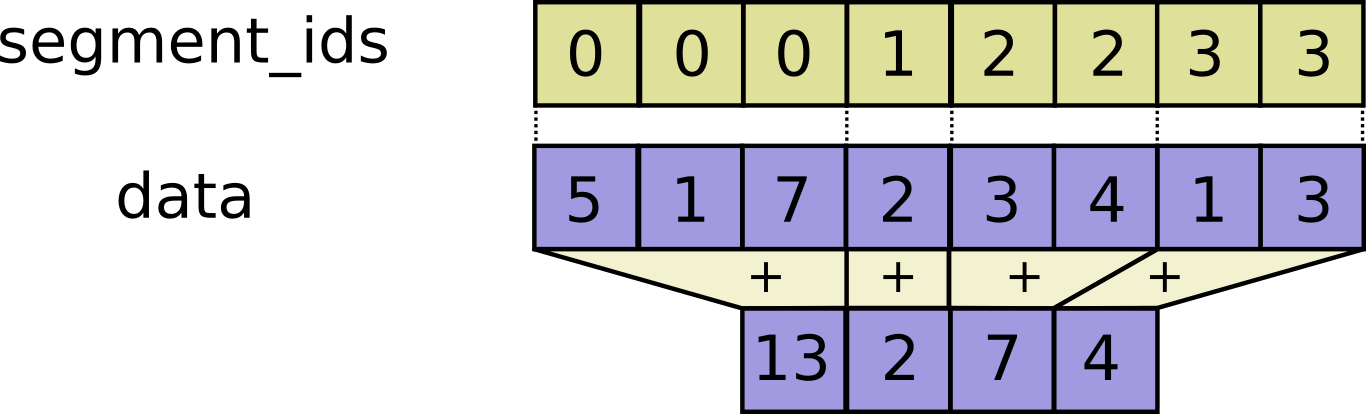# W3cubDocs

/TensorFlow Python

# tf.segment_sum

tf.segment_sum(
data,
segment_ids,
name=None
)


Defined in tensorflow/python/ops/gen_math_ops.py.

See the guide: Math > Segmentation

Computes the sum along segments of a tensor.

Read the section on segmentation for an explanation of segments.

Computes a tensor such that $$output_i = \sum_j data_j$$ where sum is over j such that segment_ids[j] == i.

If the sum is empty for a given segment ID i, output[i] = 0.#### Args:

• data: A Tensor. Must be one of the following types: float32, float64, int32, uint8, int16, int8, complex64, int64, qint8, quint8, qint32, bfloat16, uint16, complex128, half, uint32, uint64.
• segment_ids: A Tensor. Must be one of the following types: int32, int64. A 1-D tensor whose rank is equal to the rank of data's first dimension. Values should be sorted and can be repeated.
• name: A name for the operation (optional).

#### Returns:

A Tensor. Has the same type as data.